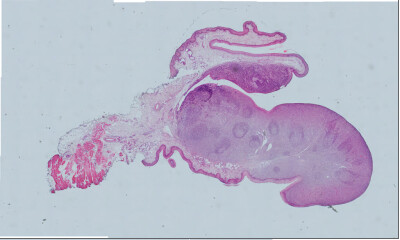# How to transfer an image buffer to matlab image matrix

hello, i want to retrieve image data from itk image, and load it in matlab.
transfer the data pixel by pixel is too slow, and there are no direct function like mxArray::new_from_buffer can do it, so i have an idea that pass the whole buffer as a 1*N array to matlab, and reshape the matrix to get a correct image.
Unfortunately the image is not correct as i expected,
the original image is 20202 * 12133 * 3:get image data in itk cpp

``````itk::Image<PixelType, Dimension>::SizeType size = resampleF->GetOutput()->GetLargestPossibleRegion().GetSize();
void * dataPtr = resampleF->GetOutput()->GetPixelContainer()->GetBufferPointer();
long long unsigned int buffer_len = 3 * size * size;
mwArray file_name(outFilename.c_str());
mwArray mwWidth(1, 1, mxUINT16_CLASS);
mwArray mwHeight(1, 1, mxUINT16_CLASS);
mwSize mdim = buffer_len;
mwArray mdisp_image(1, mdim, mxUINT8_CLASS, mxREAL);
mdisp_image.SetData((mxUint8*)dataPtr, mdim);
mwWidth.SetData(&size, 1);
mwHeight.SetData(&size, 1);
matlabProcessFuncFromDLL(mdisp_image, mwWidth, mwHeight, file_name);
``````

process the image data in matlab matlabProcessFuncFromDLL
`reshape(imgdata, [width, height, 3]) `
results like this

`reshape(imgdata, [height, width, 3])`
results

reshape and transpose it

``````reshape(imgdata, [width, height, 3])
imgdata= reshape(imgdata, [width, height, 3]);
T=affine2d([0 1 0;1 0 0;0 0 1]);
new_imgdata=imwarp(imgdata,T);
``````

result:

the image data return by GetBufferPointer structure is like this ryt?

total size is w * h * 3
is the way trans 1*N array to matlab and reshape the matrix to get a matlab image feasible, or something i understand is wrong. does Mr @matt.mccormick have any idea about this, as i notice you have a repo related to it

In ITK, channel is the fastest varying index, `rgbrgbrgb...rgb`. You have drawn channel as slowest varying `rrr...rggg...gbbb...b`. But your approach of reshaping sounds right.

1 Like

change the fast varying index to slow varying index, the output is right!
full code here:

``````chan_r = imgdata(1:3:end);
chan_g = imgdata(2:3:end);
chan_b = imgdata(3:3:end);
imgdata_combine = [chan_r, chan_g, chan_b];
mid_imgdata = reshape(imgdata_combine, [width, height, 3]);
T=affine2d([0 1 0;1 0 0;0 0 1]);
new_imgdata=imwarp(mid_imgdata,T);
``````
1 Like

channel sequence from rgbrgbrgb…rgb to rrr…rggg…gbbb…b is called convert from HWC to CHW ryt,
what if i want to do this in pure itk,
i use VectorIndexSelectionCastImageFilter first, then compose them by ComposeImageFilter like this post said, will i get a CHW image, or get the same HWC image just like split the channels before

``````// PixelType comes from completeMontage template
// template <unsigned Dimension,
//          typename PixelType,
//          typename AccumulatePixelType>
using ScalarPixelType = typename itk::NumericTraits<PixelType>::ValueType;
using ScalarImageType = itk::Image<ScalarPixelType, Dimension>;
using OriginalImageType = itk::Image<PixelType, Dimension>;
using ComposeType = itk::ComposeImageFilter<ScalarImageType, OriginalImageType>;

std::vector<typename ScalarImageType::Pointer> zImages(PixelType::Length);
for (unsigned c = 0; c < PixelType::Length; c++)
{
zImages[c]->DisconnectPipeline();
}

// compose an RGB(A) image from the filtered component images
typename ComposeType::Pointer compose = ComposeType::New();
for (unsigned c = 0; c < PixelType::Length; c++)
{
compose->SetInput(c, zImages[c]);
}
compose->Update();
``````

I think you will get the same image as before. Maybe you should combine them using tile image filter instead of ComposeImageFilter?

1 Like